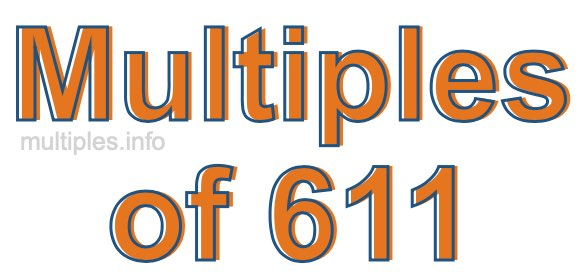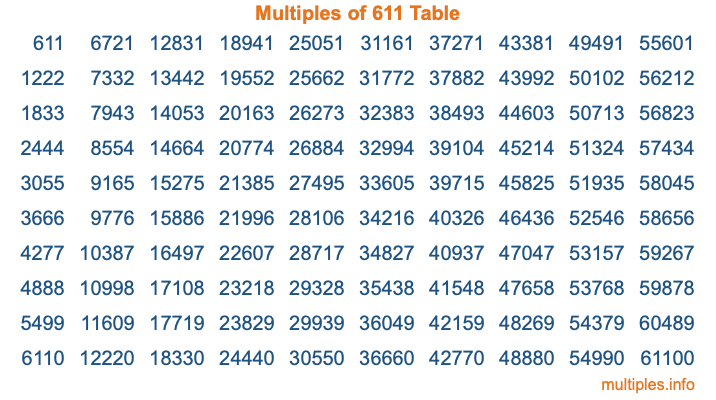Multiples of 611Welcome to the Multiples of 611 page. Here we will first teach you everything you will ever need to know about the multiples of 611, and then give you a study guide summary of everything we taught you to make sure you remember it all. Use this page to look up facts and learn information about the multiples of 611. This page will make you a multiples of six hundred eleven expert!

Definition of Multiples of 611
Multiples of 611 are all the numbers that when divided by 611 equal an integer. Each of the multiples of 611 are called a multiple. A multiple of 611 is created by multiplying 611 by an integer.

Therefore, to create a list of multiples of 611, you start with 1 multiplied by 611, then 2 multiplied by 611, then 3 multiplied by 611, and so on for as long as you want. Thus, the list of the first five multiples of 611 is 611, 1222, 1833, 2444, and 3055. To see a larger list of multiples of 611, see the printable image of Multiples of 611 further down on this page. We also have a category where you can choose any nth multiple of 611.

Multiples of 611 Checker
The Multiples of 611 Checker below checks to see if any number of your choice is a multiple of 611. In other words, it checks to see if there is any number (integer) that when multiplied by 611 will equal your number. To do that, we divide your number by 611. If the the quotient is an integer, then your number is a multiple of 611.

Is  a multiple of 611?

Least Common Multiple of 611 and ...
A Least Common Multiple (LCM) is the lowest multiple that two or more numbers have in common. This is also called the smallest common multiple or lowest common multiple and is useful to know when you are adding our subtracting fractions. Enter one or more numbers below (611 is already entered) to find the LCM.

Check out our LCM Calculator if you need more details about the Least Common Multiple or if you need the LCM for different numbers for adding and subtraction fractions.

nth Multiple of 611
As we stated above, 611 is the first multiple of 611, 1222 is the second multiple of 611, 1833 is the third multiple of 611, and so on. Enter a number below to find the nth multiple of 611.

th multiple of 611

Multiples of 611 vs Factors of 611
611 is a multiple of 611 and a factor of 611, but that is where the similarities end. All postive multiples of 611 are 611 or greater than 611. All positive factors of 611 are 611 or less than 611.

Below is the beginning list of multiples of 611 and the factors of 611 so you can compare:

Multiples of 611: 611, 1222, 1833, 2444, 3055, etc.

Factors of 611: 1, 13, 47, 611

As you can see, the multiples of 611 are all the numbers that you can divide by 611 to get a whole number. The factors of 611, on the other hand, are all the whole numbers that you can multiply by another whole number to get 611.

It's also interesting to note that if a number (x) is a factor of 611, then 611 will also be a multiple of that number (x).

Multiples of 611 vs Divisors of 611
The divisors of 611 are all the integers that 611 can be divided by evenly. Below is a list of the divisors of 611.

Divisors of 611: 1, 13, 47, 611

The interesting thing to note here is that if you take any multiple of 611 and divide it by a divisor of 611, you will see that the quotient is an integer.

Multiples of 611 Table
Below is an image of the first 100 multiples of 611 in a table. The table is in chronological order, column by column. The first column has the first ten multiples of 611, the second column has the next ten multiples of 611, and so on.The Multiples of 611 Table is also referred to as the 611 Times Table or Times Table of 611. You are welcome to print out our table for your studies.

Negative Multiples of 611
Although not often discussed or needed in math, it is worth mentioning that you can make a list of negative multiples of 611 by multiplying 611 by -1, then by -2, then by -3, and so on, to get the following list of negative multiples of 611:

-611, -1222, -1833, -2444, -3055, etc.

Multiples of 611 Summary
Below is a summary of important Multiples of 611 facts that we have discussed on this page. To retain the knowledge on this page, we recommend that you read through the summary and explain to yourself or a study partner why they hold true.

There are an infinite number of multiples of 611.

A multiple of 611 divided by 611 will equal a whole number.

611 divided by a factor of 611 equals a divisor of 611.

The nth multiple of 611 is n times 611.

The largest factor of 611 is equal to the first positive multiple of 611.

611 is a multiple of every factor of 611.

611 is a multiple of 611.

A multiple of 611 divided by a divisor of 611 equals an integer.

611 divided by a divisor of 611 equals a factor of 611.

Any integer times 611 will equal a multiple of 611.

Multiples of a Number
Here you can get the multiples of another number, all with the same attention to detail as we did for multiples of 611 on this page.

Multiples of
Multiples of 612
Did you find our page about multiples of six hundred eleven educational? Do you want more knowledge? Check out the multiples of the next number on our list!